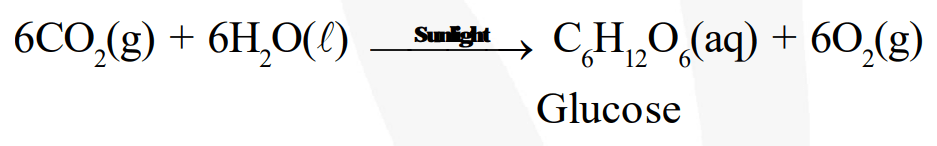# What does one mean by exothermic and endothermic reactions?Question.
What does one mean by exothermic and endothermic reactions? Give examples.

solution:
Chemical reactions that release energy in the form of heat, light, or sound are called exothermic reactions. Example: Reaction of sodium and chlorine to yield common salt

$\mathrm{Na}(\mathrm{s})+\frac{\mathbf{1}}{\mathbf{2}} \mathrm{Cl}_{2}(\mathrm{~s}) \rightarrow \mathrm{NaCl}(\mathrm{s})+411 \mathrm{~kJ}$ of energy

In other words, combination reactions are exothermic. Reactions that absorb energy or require energy in order to proceed are called endothermic reactions. For example: In the process of photosynthesis, plants use the energy from the sun to convert carbon dioxide and water to glucose and oxygen.